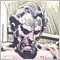# Struggeling to go back 2 bars.23

Hi,

First a confession... I am not good at programming :-)

I hope that someone can / will help me.

I have some code to make Heiken Ashi Candles, now I would like to put a condition for 2 candles back. But I can't seem to get it right.

Here is the Code:

```int OnCalculate(const int rates_total,
const int prev_calculated,
const datetime &time[],
const double &open[],
const double &high[],
const double &low[],
const double &close[],
const long &tick_volume[],
const long &volume[],
{
int    i,pos;
double haOpen,haHigh,haLow,haClose;
//---
if(rates_total<=10)
return(0);
//--- counting from 0 to rates_total
ArraySetAsSeries(ExtLowHighBuffer,false);
ArraySetAsSeries(ExtHighLowBuffer,false);
ArraySetAsSeries(ExtOpenBuffer,false);
ArraySetAsSeries(ExtCloseBuffer,false);
ArraySetAsSeries(open,false);
ArraySetAsSeries(high,false);
ArraySetAsSeries(low,false);
ArraySetAsSeries(close,false);
//--- preliminary calculation
if(prev_calculated>1)
pos=prev_calculated-1;
else
{
//--- set first candle
if(open<close)
{
ExtLowHighBuffer=low;
ExtHighLowBuffer=high;
}
else
{
ExtLowHighBuffer=high;
ExtHighLowBuffer=low;
}
ExtOpenBuffer=open;
ExtCloseBuffer=close;
//---
pos=1;
}
//--- main loop of calculations
for(i=pos; i<rates_total; i++)
{
haOpen=(ExtOpenBuffer[i-1]+ExtCloseBuffer[i-1])/2;
haClose=(open[i]+high[i]+low[i]+close[i])/4;
haHigh=MathMax(high[i],MathMax(haOpen,haClose));
haLow=MathMin(low[i],MathMin(haOpen,haClose));
if(haOpen<haClose)
{
ExtLowHighBuffer[i]=haLow;
ExtHighLowBuffer[i]=haHigh;
}
else
{
ExtLowHighBuffer[i]=haHigh;
ExtHighLowBuffer[i]=haLow;
}
ExtOpenBuffer[i]=haOpen;
ExtCloseBuffer[i]=haClose;

if (open[i-2] > close[i-2]){
Comment ("Bearish");
}
}
//--- done

return(rates_total);
}```

so the error is at:

```if (open[i-2] > close[i-2]){
Comment ("Bearish");
}```

I have tried to add to rates_total, but no success.

How could I acomplish this?

Kind Regards3297

so the error is at:

Think about the variable i and what it's values are. What happens when i = 1? You are trying to find the open[-1] and close[-1]. That's not going to fly.

A quick fix is:

```if (i > 1 && open[i-2] > close[i-2]){
Comment ("Bearish");
}
```23

Anthony Garot:

Think about the variable i and what it's values are. What happens when i = 1? You are trying to find the open[-1] and close[-1]. That's not going to fly.

A quick fix is:

Thank you Very much!! so simple.20233